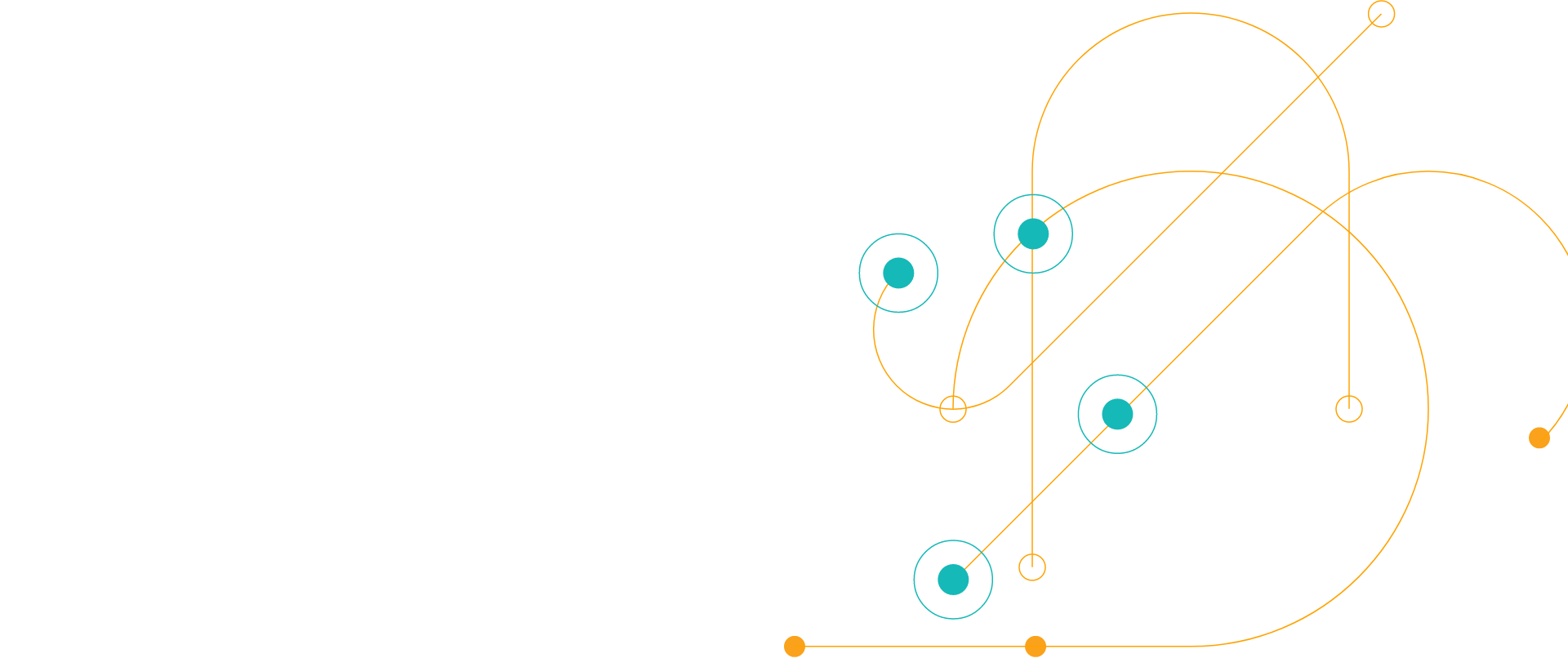# The Chef Automate Guide to PCI DSS Compliance

Back to White Papers

This guide covers the 12 PCI DSS security requirements that apply to all system components. For each requirement, this guide illustrates how to use existing InSpec controls, or create new controls, to verify compliance.

Read the Chef Automate Guide to PCI DSS Compliance White Paper

<div class='-tac -pb2'> <div class='svgi -c-magenta -mb1'> <svg xmlns='http://www.w3.org/2000/svg' width='178.099' height='129.473' viewBox='0 0 178.099 129.473'><defs><style>.a{fill:none;stroke:#d91683;stroke-miterlimit:10;stroke-width:2px;}</style></defs><g transform='translate(-964 -388)'><g transform='translate(2053.531 592.899)'><path class='a' d='M-1001.416-112.527h0a15.244,15.244,0,0,0,15.242-15.244v-7.543a2.33,2.33,0,0,1,2.332-2.33h1.15a7.628,7.628,0,0,1,7.628,7.627v17.49h12.3a7.469,7.469,0,0,1,7.469,7.469,7.481,7.481,0,0,1-.291,2.065l-5.254,18.267a7.47,7.47,0,0,1-7.178,5.4h-23.82'/><path class='a' d='M-1016.167-89.561v-22.586a5.337,5.337,0,0,1,5.337-5.339h4.075a5.339,5.339,0,0,1,5.339,5.339v36.721'/><circle class='a' cx='2.418' cy='2.418' r='2.418' transform='translate(-1027.341 -153.295)'/><circle class='a' cx='3.089' cy='3.089' r='3.089' transform='translate(-932.889 -108.485)'/><circle class='a' cx='3.089' cy='3.089' r='3.089' transform='translate(-1045.892 -148.25)'/><line class='a' transform='translate(-1032.363 -112.148)'/><line class='a' x1='7.549' transform='translate(-1024.031 -112.148)'/><line class='a' x1='11.31' transform='translate(-1027.341 -79.57)'/><line class='a' y1='30.648' transform='translate(-982.378 -199.62)'/><line class='a' y1='9.209' transform='translate(-987.339 -204.899)'/><line class='a' x2='14.429' transform='translate(-945.128 -133.443)'/><line class='a' x2='16.313' transform='translate(-1029.224 -133.443)'/><line class='a' x1='17.459' y1='17.459' transform='translate(-1024.252 -173.651)'/><line class='a' y1='17.459' x2='17.459' transform='translate(-954.858 -173.657)'/><line class='a' x2='15.442' transform='translate(-926.874 -133.443)'/><circle class='a' cx='5.045' cy='5.045' r='5.045' transform='translate(-924.198 -158.341)'/><circle class='a' cx='3.93' cy='3.93' r='3.93' transform='translate(-956.811 -203.551)'/><path class='a' d='M-1002.58-130.114a21.3,21.3,0,0,1,21.3-21.295,21.3,21.3,0,0,1,21.295,21.295'/><path class='a' d='M-1007.275-130.674a25.99,25.99,0,0,1,25.99-25.99,25.99,25.99,0,0,1,25.99,25.99'/><path class='a' d='M-1022.911-112.861a32.811,32.811,0,0,1-32.81,32.81,32.811,32.811,0,0,1-32.81-32.81'/><circle class='a' cx='25.308' cy='25.308' r='25.308' transform='translate(-1081.029 -137.456)'/><path class='a' d='M-1065.55-107.007l9.829,7.8,9.829-7.8'/><line class='a' y2='25.892' transform='translate(-1055.721 -125.094)'/><circle class='a' cx='0.565' cy='0.565' r='0.565' transform='translate(-969.36 -164.564)'/><circle class='a' cx='0.565' cy='0.565' r='0.565' transform='translate(-945.692 -110.858)'/><line class='a' x1='3.557' y2='3.557' transform='translate(-939.276 -184.213)'/><line class='a' x1='9.946' y2='9.946' transform='translate(-934.773 -186.311)'/><line class='a' x1='9.946' y2='9.946' transform='translate(-937.266 -173.945)'/><line class='a' x1='9.946' y1='9.946' transform='translate(-1039.929 -186.311)'/><line class='a' x1='9.946' y1='9.946' transform='translate(-1037.436 -173.945)'/><circle class='a' cx='2.605' cy='2.605' r='2.605' transform='translate(-1012.516 -82.175)'/></g></g></svg> </div> <h3>Thank You</h3> <p>If your download hasn't started in 5 seconds please</p> <p><a data-sf-ec-immutable='' href='https://d1l5pp53ux74mz.cloudfront.net/docs/default-source/whitepapers/guidetopcidsscompliance.pdf?sfvrsn=cafa28b9_4' class='-i-next' target='_blank' rel='nofollow'>Click here to download </a></p> </div> <script>document.getElementsByClassName('js-form-title').style.display='none';var win=window.open('https://d1l5pp53ux74mz.cloudfront.net/docs/default-source/whitepapers/guidetopcidsscompliance.pdf?sfvrsn=cafa28b9_4', '_blank');win.focus();</script><script>if (typeof (window.dataLayer) !== 'undefined') { window.dataLayer = window.dataLayer || []; dataLayer.push({'event': 'custom.successMessageShown'}); } </script>